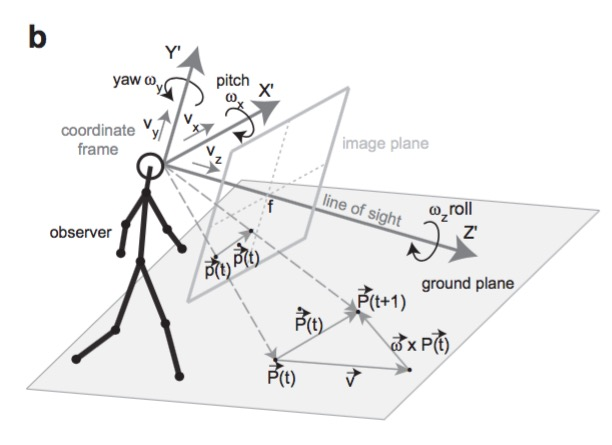# Parameters for action

science
Published

January 31, 2019

Several years ago, Florian Raudies, Swapnaa Jayaraman and I published a paper where we simulated the optic flow that infants would experience in different head/body postures. We computed cyclopian (one-eyed) flow on the basis of this schematic:Here, the key parameters were the instantaneous translation $$(v_x{}, v_y{}, v_z{})$$ and rotation $$(\omega_{x}, \omega_y{}, \omega_z{})$$ of the planar retina. Coupled with the optic flow equation,

$$\begin{pmatrix}\dot{x} \\ \dot{y}\end{pmatrix}=\frac{1}{z} \begin{pmatrix}-f & 0 & x\\ 0 & -f & y \end{pmatrix} \begin{pmatrix}{v_x{}}\\ {v_y{}} \\{v_z{}}\end{pmatrix}+ \frac{1}{f} \begin{pmatrix} xy & -(f^2+x^2) & fy\\ f^2+y^2 & -xy & -fy \end{pmatrix} \begin{pmatrix} \omega_{x}\\ \omega_{y}\\ \omega_{z} \end{pmatrix}$$

we were able to simulate the perceptual effects of postural geometry: Changes in eye height and forward translational speed that would occur when a child changed from crawling to walking altered the pattern of retinal flow $$(\dot{x}, \dot{y})$$ in interesting ways.

This work has lain dormant for a few years, but I now want to pick it back up. In short, there are a handful of perception/action systems that provide the nervous system with deterministic, causal information about the effects of different actions. These must be important for development.

For the next step, I’m looking for a concise, but thorough parameterization of body posture that includes the eyes, head, torso, arms, and legs. Here’s a sketch of what I have in mind for the upper parts body that have the greatest impact on the direction of visual fixation:

Body part Parameter(s)
Eyes ${rx}, {ry}, {lx}, {lx}$
Head $$\theta_x{}, \theta_y{}, \theta_z{}$$
Torso $_x{}, _y{}, _z{}$

Coupled with the distance between the eyes, $$i$$, the radial distance to the head’s center of rotation, $$h$$, and the distance from the head’s center of rotation to the torso’s center of rotation, $$t$$, we can compute the effects of eye, head, and torso movement on visual motion at the two retinae. Now, if the visual signals from eye vs. head vs. torso can be distinguished, then these could couple with other proprioceptive (muscle, tendon, cutaneous) signals to provide a powerful set of sensory signals that are directly caused by eye, head, and torso motion. See this earlier post for a causal graph that elaborates on this point. I’ll discuss why I think there are visual differences in the effects of eye and head motion in a future post.

My next step is to ask my colleagues in kinesiology if there is a canonical parameterization of body position that I can build upon. If you know of one, let me know.